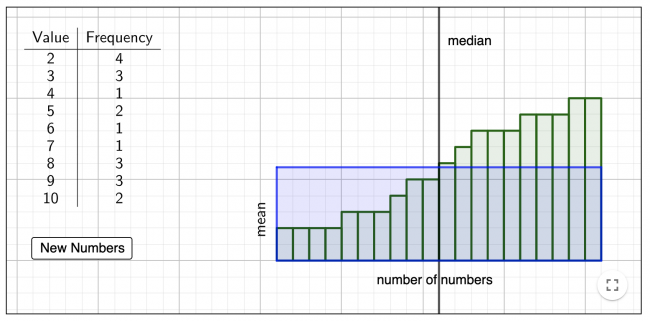# Visualize the Mean and Median

Preview:This activity lets students visualize the relationship between a table of values showing the frequency of different numbers and the mean and median of that set of data.

Technology
Big Idea:
Grade 8: Analyzing data by determining averages is one way to make sense of large data sets and enables us to compare and interpret.
Curricular Competency:
Reasoning and analyzing: Use reasoning to explore and make connections
Reasoning and analyzing: Use technology to explore mathematics
Understanding and solving: Visualize to explore mathematical concepts
Communicating and representing: Use mathematical vocabulary and language to contribute to mathematical discussions